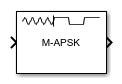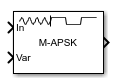# M-APSK Demodulator Baseband

M-ary amplitude phase shift keying (APSK) demodulation

• Library:
• Communications Toolbox / Modulation / Digital Baseband Modulation / APM

•## Description

The M-APSK Demodulator Baseband block demodulates a baseband representation of an M-ary amplitude phase shift keying (APSK) modulated signal. M is the Modulation Order for M-APSK. For a description of M-APSK demodulation, see APSK Hard Demodulation and APSK Soft Demodulation.

Note

M-APSK Demodulator Baseband specifically applies to multiple ring PSK constellations. For a single ring PSK constellation, use M-PSK Demodulator Baseband.

This icon shows the block with all ports enabled:## Ports

### Input

expand all

APSK modulated signal, specified as a scalar, vector, or matrix. When this input is a matrix, each column is treated as an independent channel. This port is unnamed until the `Var` port is enabled.

Data Types: `double` | `single`
Complex Number Support: Yes

Noise variance, specified as a positive scalar or vector of positive values. When the noise variance or signal power result in computations involving extreme positive or negative magnitudes, see APSK Soft Demodulation for demodulation decision type considerations.

#### Dependencies

To enable this port, set Noise variance source to ```Input port```.

Data Types: `double` | `single`

### Output

expand all

Demodulated signal, returned as a scalar, vector, or matrix. The data type and dimensions of the demodulated signal depend on the values specified by the Output type and Decision type parameters. This port is unnamed on the block.

Output type Decision typeDemodulated Signal DescriptionDimensions of Demodulated Signal
`Integer`Demodulated integer values in the range [0, (M – 1)]The output signal has the same dimensions as the input signal.
`Bit``Hard decision`Demodulated bitsThe number of rows in the output signal is log2(M) times the number of rows in the input signal. Each demodulated symbol is mapped to a group of log2(M) elements in a column, where the first element represents the MSB and the last element represents the LSB.
```Log-likelihood ratio```Log-likelihood ratio value for each bit
```Approximate log-likelihood ratio```Approximate log-likelihood ratio value for each bit

M is the Modulation Order for M-APSK.

Use Output data type to specify the output data type.

Data Types: `single` | `double`

## Parameters

expand all

Constellation points per PSK ring, specified as a vector with more than one element. Each vector element indicates the number of constellation points in its corresponding PSK ring. The first element corresponds to the innermost circle, and so on until the last element, which corresponds to the outermost circle. The sum of the elements in Constellation points per circle determines the modulation order. Element values must be multiples of four, and the modulation order must be a power of two.

Example: `[4,12,16]` specifies a three PSK ring constellation with a modulation order of 32.

Radius per PSK ring, specified as a vector with the same length as Constellation points per circle. Each vector element indicates the radius of its corresponding PSK ring. The first element corresponds to the innermost circle, and so on until the last element, which corresponds to the outermost circle. These element values must be positive values arranged in increasing order.

Example: `[0.5,1,2]` defines radii for three constellation PSK rings. The inner ring has a radius of 0.5, the second ring has a radius of 1.0, and the outer ring has a radius of 2.0.

Phase offset per PSK ring in radians, specified as a scalar or vector with the same length as Constellation points per circle. Each vector element indicates the phase offset of its corresponding PSK ring. The first element corresponds to the innermost circle, and so on until the last element, which corresponds to the outermost circle. The Phase offset of each circle (rad) can be a scalar only if all the elements of Constellation points per circle are the same value.

Example: `[pi/4,pi/12,pi/16]` defines phase offsets for three constellation PSK rings. The inner ring has a phase offset of π/4, the second ring has a phase offset of π/12, and the outer ring has a phase offset of π/16.

Symbol mapping, specified as one of the following:

• `Contourwise-gray` — Uses Gray mapping along the contour in the phase dimension for each PSK ring.

• `Gray` — Uses Gray mapping along the contour in both the amplitude and phase dimensions. For Gray symbol mapping, all elements in Constellation points per circle must be equal, and all elements in Phase offset of each circle (rad) must be equal. For a description of the Gray mapping used, see .

• `User-defined` — See Custom symbol mapping.

The default symbol mapping depends on the Constellation points per circle and Phase offset of each circle (rad) parameters. When all elements in Constellation points per circle are equal, and all elements in Phase offset of each circle (rad) are equal, the default is `Gray`. For all other cases, the default is `Contourwise-gray`.

Custom symbol mapping, specified as an integer vector. This vector must consist of M unique elements with values in the range [0, (M – 1)], where M is the Modulation Order for M-APSK. The first element in Custom symbol mapping corresponds to the constellation point in the first quadrant of the innermost circle, with subsequent elements positioned counterclockwise around the PSK rings.

Example: The default value, `[0,4,12,8,1,3,2,6,7,5,13,15,14,10,11,9]`, specifies contourwise-gray symbol mapping. The distribution of constellation points is nonuniform on all contours.

#### Dependencies

To enable this parameter, set Symbol mapping to `User-defined`.

Output type, specified as `Integer` or `Bit`.

Data Types: `char` | `string`

Demodulation decision type, specified as ```Hard decision```, `Log-likelihood ratio`, or `Approximate log-likelihood ratio`. See APSK Soft Demodulation for algorithm selection considerations.

#### Dependencies

This parameter applies only when Output type is set to `Bit`.

Noise variance source, specified as:

• `Property` — The noise variance is set using the Noise variance parameter.

• `Input port` — The noise variance is set using the Var input port.

#### Dependencies

This parameter applies only when Decision type is set to either `Log-likelihood ratio` or `Approximate log-likelihood ratio`.

Noise variance, specified as a positive scalar or vector of positive values.

• When specified as a scalar, that value is used on all elements in the input signal.

• When specified as a vector, the vector length must be equal to the number of columns in the input signal. Each noise variance vector element is applied to its corresponding column in the input signal.

When the noise variance or signal power result in computations involving extreme positive or negative magnitudes, see APSK Soft Demodulation for Decision type specification considerations.

#### Dependencies

This parameter applies only when Noise variance source is set to `Property` and Decision type is set to either `Log-likelihood ratio` or `Approximate log-likelihood ratio`.

Data Types: `double`

Output data type, specified as one of the acceptable values from this table. Acceptable Output data type values depend on the Output type and Decision type parameter values.

Output typeDecision typeOutput data type Options
`Integer`Not applicable`double`, `single`, `int8`, `uint8`, `int16`, `uint16`, `int32`, or `uint32`
`Bit``Hard decision``double`, `single`, `int8`, `uint8`, `int16`, `uint16`, `int32`, `uint32`, or `logical`
`Log-likelihood ratio` or ```Approximate log-likelihood ratio```The output signal is the same data type as the input signal.

#### Dependencies

This parameter applies only when Output type is set to `Integer` or when Output type is set to `Bit` and Decision type is set to ```Hard decision```.

Type of simulation to run, specified as:

• `Code generation` –– Simulate the model using generated C code. The first time you run a simulation, Simulink® generates C code for the block. The C code is reused for subsequent simulations as long as the model does not change. This option requires additional startup time.

• `Interpreted execution` –– Simulate the model using the MATLAB® interpreter. This option shortens startup time. In `Interpreted execution` mode, you can debug the source code of the block.

For information on execution speed, see Tips.

## Block Characteristics

 Data Types `Boolean` | `double` | `integer` | `single` Multidimensional Signals `yes` Variable-Size Signals `no`

expand all

## Tips

• For faster execution of the M-APSK Demodulator Baseband block, set the Simulate using parameter to:

• `Code generation` when using hard decision demodulation.

• `Interpreted execution` when using soft decision demodulation.

 Sebesta, J. “Efficient Method for APSK Demodulation.” Selected Topics on Applied Mathematics, Circuits, Systems, and Signals (P. Pardalos, N. Mastorakis, V. Mladenov, and Z. Bojkovic, eds.). Vouliagmeni, Athens, Greece: WSEAS Press, 2009.

 Liu, Z., Q. Xie, K. Peng, and Z. Yang. "APSK Constellation with Gray Mapping." IEEE Communications Letters. Vol. 15, Number 12, December 2011, pp. 1271–1273.